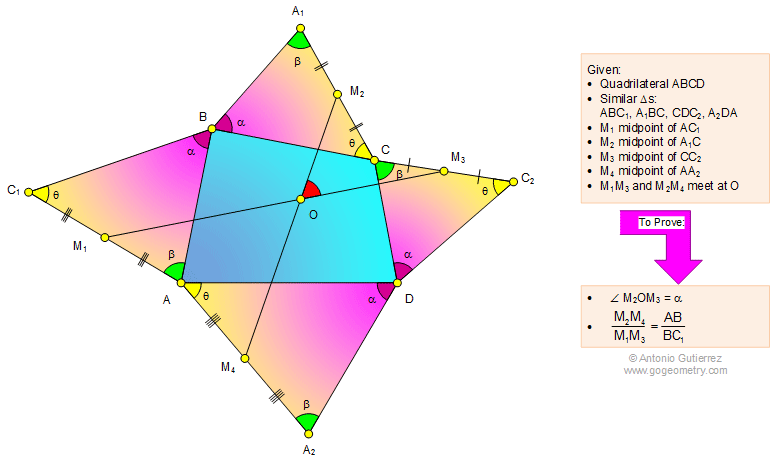# Problem 1119: Quadrilateral, Similar Triangles, Midpoints, Equal Ratio, Angles. Level: High School, SAT Prep, College Geometry

< PREVIOUS PROBLEM  |  NEXT PROBLEM >

 The figure below shows a quadrilateral ABCD with similar triangles ABC1, A1BC, CDC2, and A2DA. M1, M2, M3, and M4 are the midpoints of AC1, A1C, CC2, and AA2, respectively. M1M3 and M2M4 meet at O. Prove that: (1) angle M2OM3 = angle ABC1; (2).Selected Topics

 Home | Search | Geometry | Problems | All Problems | Open Problems | Visual Index | 1111-1120 | Triangle | Quadrilateral | Similarity, Ratio, Proportion | Midpoint | Angles | Email | Post a comment or solution | By Antonio GutierrezLast updated: May 6, 2015